# CNN眼中的世界：利用Keras解释CNN的滤波器

## 文章信息

### 使用Keras探索卷积网络的滤波器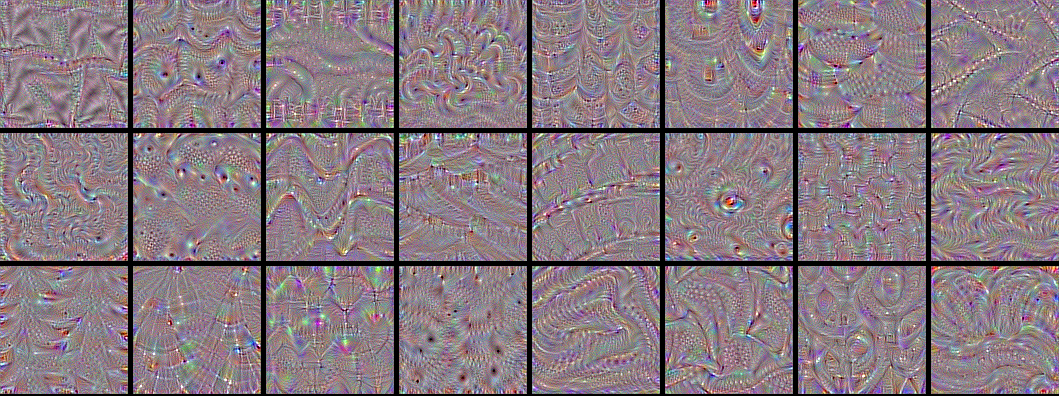VGG-16又称为OxfordNet，是由牛津视觉几何组（Visual Geometry Group）开发的卷积神经网络结构。该网络赢得了ILSVR（ImageNet）2014的冠军。时至今日，VGG仍然被认为是一个杰出的视觉模型——尽管它的性能实际上已经被后来的Inception和ResNet超过了。

Lorenzo Baraldi将Caffe预训练好的VGG16和VGG19模型转化为了Keras权重文件，所以我们可以简单的通过载入权重来进行实验。该权重文件可以在这里下载。国内的同学需要自备梯子。（这里是一个网盘保持的vgg16：http://files.heuritech.com/weights/vgg16_weights.h5赶紧下载，网盘什么的不知道什么时候就挂了。）

``````from keras.models import Sequential
from keras.layers import Convolution2D, ZeroPadding2D, MaxPooling2D

img_width, img_height = 128, 128

# build the VGG16 network
model = Sequential()
first_layer = model.layers[-1]
# this is a placeholder tensor that will contain our generated images
input_img = first_layer.input

# build the rest of the network

# get the symbolic outputs of each "key" layer (we gave them unique names).
layer_dict = dict([(layer.name, layer) for layer in model.layers])
``````

``````import h5py

weights_path = 'vgg16_weights.h5'

f = h5py.File(weights_path)
for k in range(f.attrs['nb_layers']):
if k >= len(model.layers):
# we don't look at the last (fully-connected) layers in the savefile
break
g = f['layer_{}'.format(k)]
weights = [g['param_{}'.format(p)] for p in range(g.attrs['nb_params'])]
model.layers[k].set_weights(weights)
f.close()
``````

``````from keras import backend as K

layer_name = 'conv5_1'
filter_index = 0  # can be any integer from 0 to 511, as there are 512 filters in that layer

# build a loss function that maximizes the activation
# of the nth filter of the layer considered
layer_output = layer_dict[layer_name].output
loss = K.mean(layer_output[:, filter_index, :, :])

# compute the gradient of the input picture wrt this loss

# normalization trick: we normalize the gradient

# this function returns the loss and grads given the input picture
``````

``````import numpy as np

# we start from a gray image with some noise
input_img_data = np.random.random((1, 3, img_width, img_height)) * 20 + 128.
# run gradient ascent for 20 steps
for i in range(20):
``````

``````from scipy.misc import imsave

# util function to convert a tensor into a valid image
def deprocess_image(x):
# normalize tensor: center on 0., ensure std is 0.1
x -= x.mean()
x /= (x.std() + 1e-5)
x *= 0.1

# clip to [0, 1]
x += 0.5
x = np.clip(x, 0, 1)

# convert to RGB array
x *= 255
x = x.transpose((1, 2, 0))
x = np.clip(x, 0, 255).astype('uint8')
return x

img = input_img_data
img = deprocess_image(img)
imsave('%s_filter_%d.png' % (layer_name, filter_index), img)
``````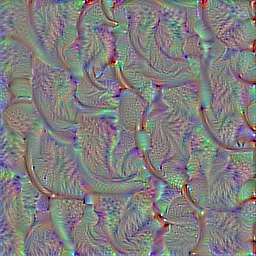### 可视化所有的滤波器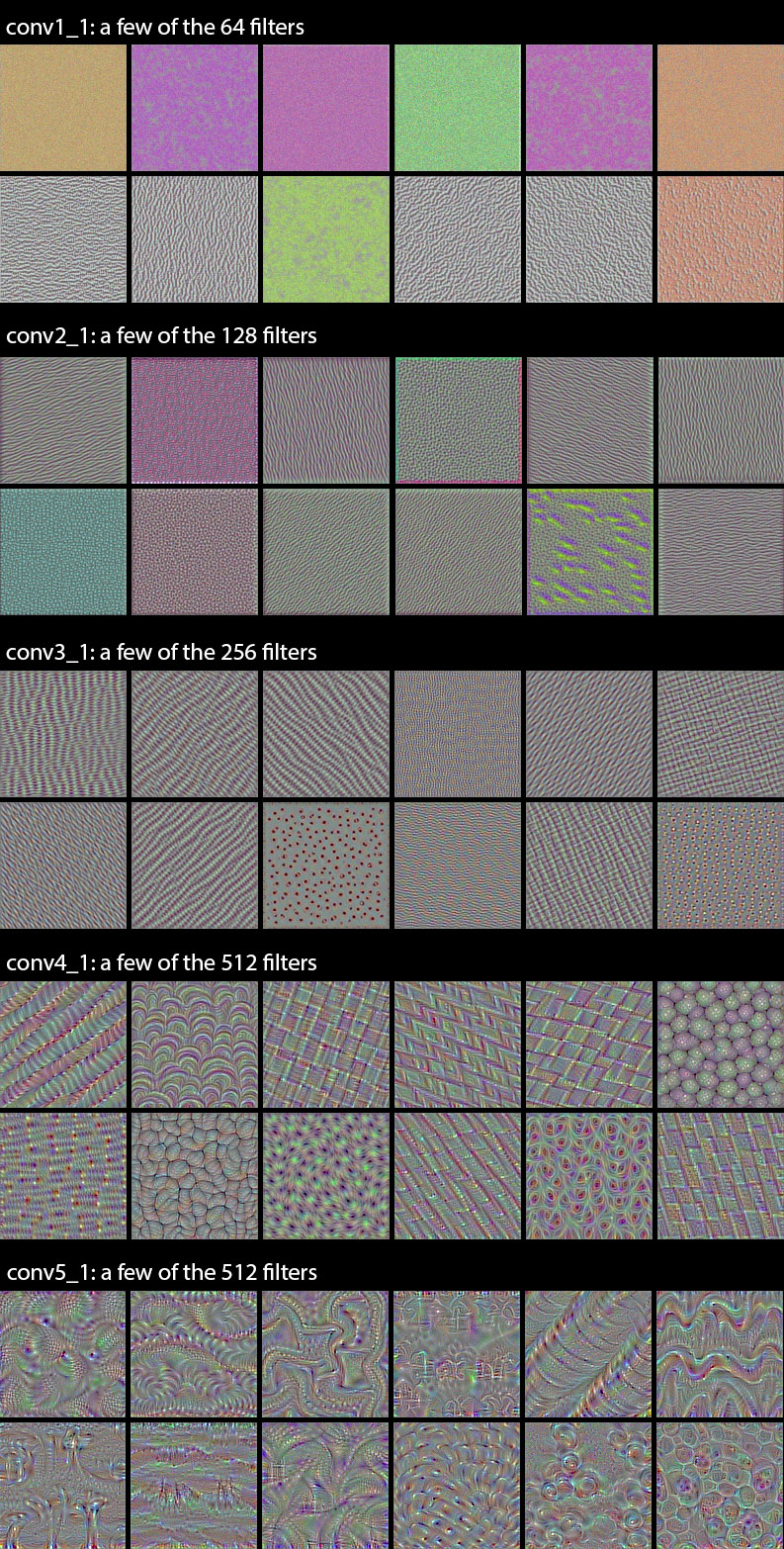### Deep Dream（nightmare）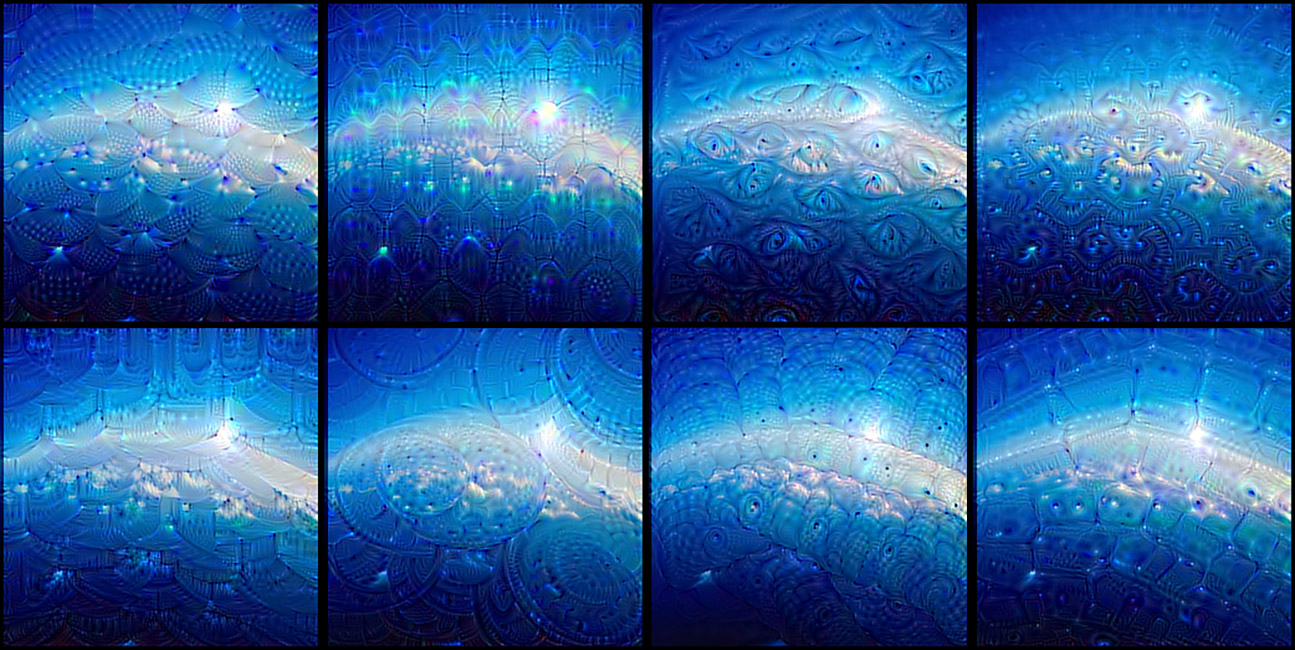### 愚弄神经网络

``````layer_output = model.layers[-1].get_output()
loss = K.mean(layer_output[:, output_index])
``````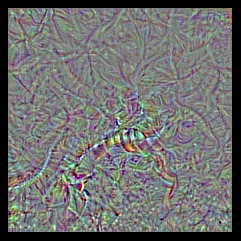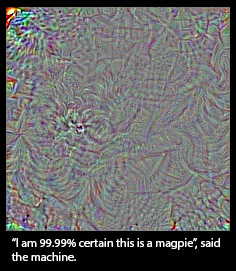OK，我们的网络认为是喜鹊的东西看起来完全不是喜鹊，往好了说，这个图里跟喜鹊相似的，也不过就是一些局部的纹理，如羽毛，嘴巴之类的。那么，这就意味着卷积神经网络是个很差的工具吗？当然不是，我们按照一个特定任务来训练它，它就会在那个任务上表现的不错。但我们不能有网络“理解”某个概念的错觉。我们不能将网络人格化，它只是工具而已。比如一条狗，它能识别其为狗只是因为它能以很高的概率将其正确分类而已，而不代表它理解关于“狗”的任何外延。

### 革命尚未成功，同志仍需努力

@fchollet, 2016年1月